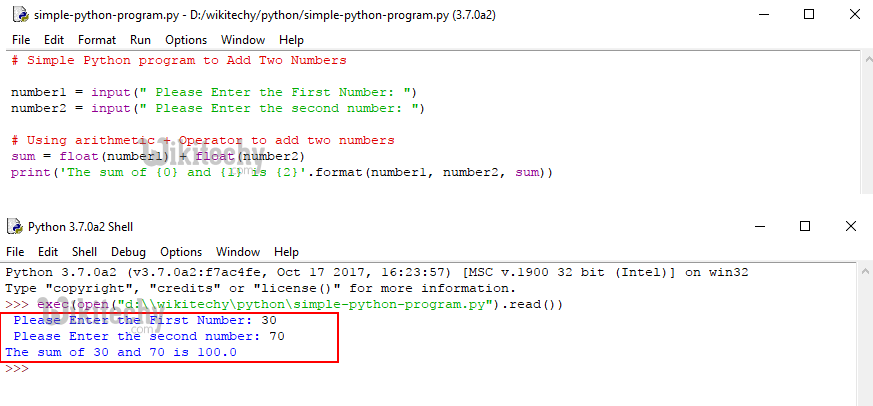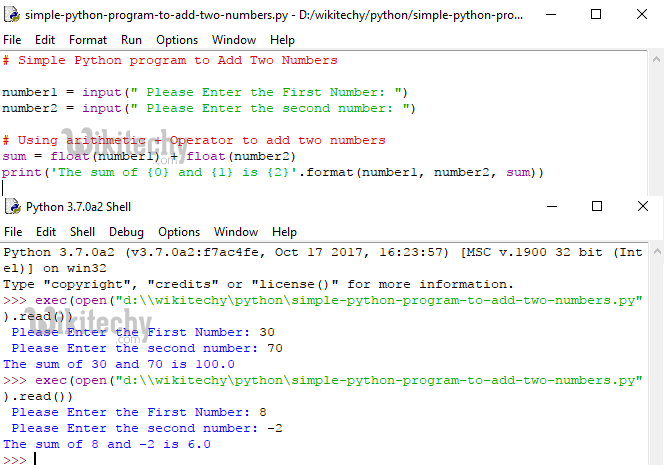# python tutorial - Simple Python Program - learn python - python programming

• To write a Simple Python Program with example. In order to show, we are going to add two numbers using Arithmetic Operators

## Simple Python Program to add Two numbers

• This simple Python program allows the user to enter two values and then add those two numbers and assign the total to variable sum.

## OutputLearn Python - Python tutorial - simple python program - Python examples - Python programs

## Analysis

• Following statements ask the user to enter two integer numbers and stores the user entered values in variables number 1 and number 2
• Next line, we used Python Arithmetic Operators ‘+’ to add number1 and number2 and then assigned that total to sum.
• From the below statement you can observe that, we used float type cast to convert the user input values to float.
• This is because, by default user entered values will be of string type and if we use the + operator in between two string values python will concat those two values instead of adding them
• Following print statement will print the sum variable as Output (30 + 70= 100).
• This program worked well while adding two positive integers, How about adding positive and negative integer? Let us seeLearn Python - Python tutorial - Simple Python Program to add Two numbers - Python examples - Python programs

• Alternative way of writing the above code is:

NOTE: Above code will restrict the user, Not to enter string values as input